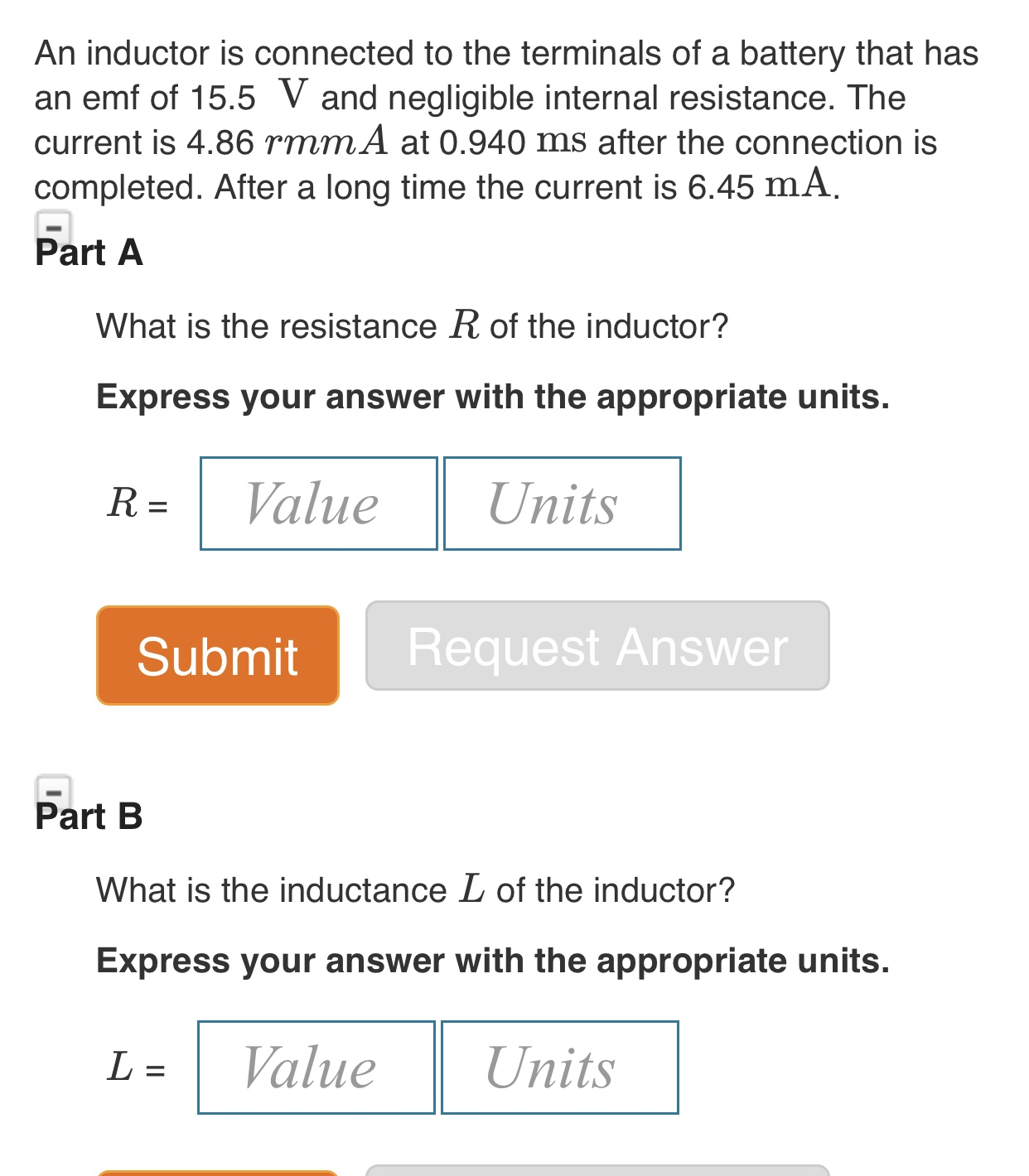# An inductor is connected to the terminals of a battery that hasan emf of 15.5 V and negligible internal resistance. Thecurrent is 4.86 rmmA at 0.940 ms after the connection iscompleted. After a long time the current is 6.45 mAPart AWhat is the resistance R of the inductor?Express your answer with the appropriate units.ValueUnitsR =Request AnswerSubmitPart BWhat is the inductance L of the inductor?Express your answer with the appropriate units.ValueUnitsL =

Question
2 views

An inductor is connected to the terminals of a  battery that hasn’t an emf of 15.5 V and negligible internal resistance. The current is 4.86 rmmA at 0.940 ma after the connection Ian completed. After a long time the current is 6.45mA.

A) What is the resistance R of the inductor?

R = _______ units _____

B) What is the inductance L of the inductor?

L = _________ units ____help_outlineImage TranscriptioncloseAn inductor is connected to the terminals of a battery that has an emf of 15.5 V and negligible internal resistance. The current is 4.86 rmmA at 0.940 ms after the connection is completed. After a long time the current is 6.45 mA Part A What is the resistance R of the inductor? Express your answer with the appropriate units. Value Units R = Request Answer Submit Part B What is the inductance L of the inductor? Express your answer with the appropriate units. Value Units L = fullscreen
check_circle

Step 1

a)

The required resis...

### Want to see the full answer?

See Solution

#### Want to see this answer and more?

Solutions are written by subject experts who are available 24/7. Questions are typically answered within 1 hour.*

See Solution
*Response times may vary by subject and question.
Tagged in

### Alternating Current